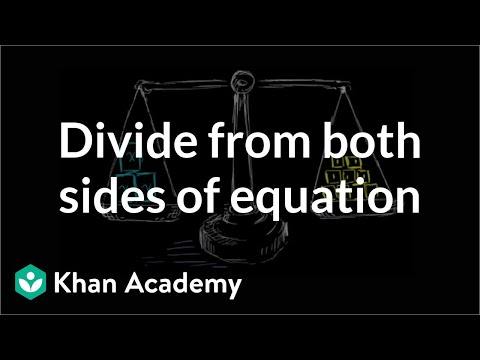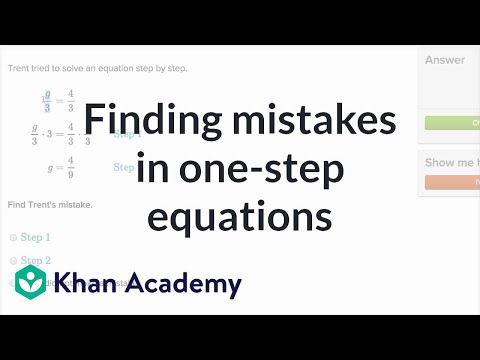Video

# One-step addition & subtraction equations (Full video)

Khan Academy

## Supporting grades: 6

Description: Learn how to solve one-step addition and subtraction equations by adding or subtracting the same thing from both sides of the equation. So let's say that you have x plus seven is equal to ten, and I want to solve for x. All its saying is something plus seven is equal to ten, and you might be able to figure that out in your head, but if you want to do it a little bit more systematically, you're like well just all I want on the left hand side is an x. Well if all I want on the left hand side is an x I'd want to get rid of the seven.

You must log inorsign upif you want to:*

*Teacher Advisor is 100% free.

### Other videos you might be interested in### Dividing both sides of an equation (Full video)

#### Khan Academy